3.MD.7.A.-Test1B Find the area of a rectangle with whole-number side lengths
 Name:    3.MD.7.A.-Test1B Find the area of a rectangle with whole-number side lengths

Multiple Choice
Identify the choice that best completes the statement or answers the question.

1.

How many tiles would it take to cover the rectangle?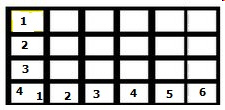a. 25 c. 16 b. 24 d. 20

2.

How many tiles would it take to cover the rectangle?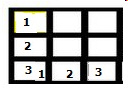a. 9 c. 10 b. 6 d. 8

3.

How many tiles would it take to cover the rectangle?a. 12 c. 10 b. 16 d. 8

4.

How many bricks would it take to build the side of this house?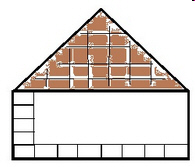a. 13 c. 40 b. 26 d. 32

5.

How many bricks would it take to build the side of this house?a. 13 c. 40 b. 26 d. 32

6.

Bob was paving his patio.  How many square units will he need to completely pave it?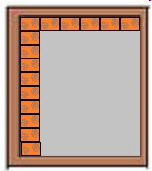a. 60 c. 27 b. 54 d. 16

7.

How many tiles would it take to cover the rectangle?a. 10 c. 12 b. 20 d. 16

8.

Which equation best describes the formula for the area of this rectangle?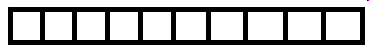a. 10 c. 1 x 10 b. 10 x 10 d. 20